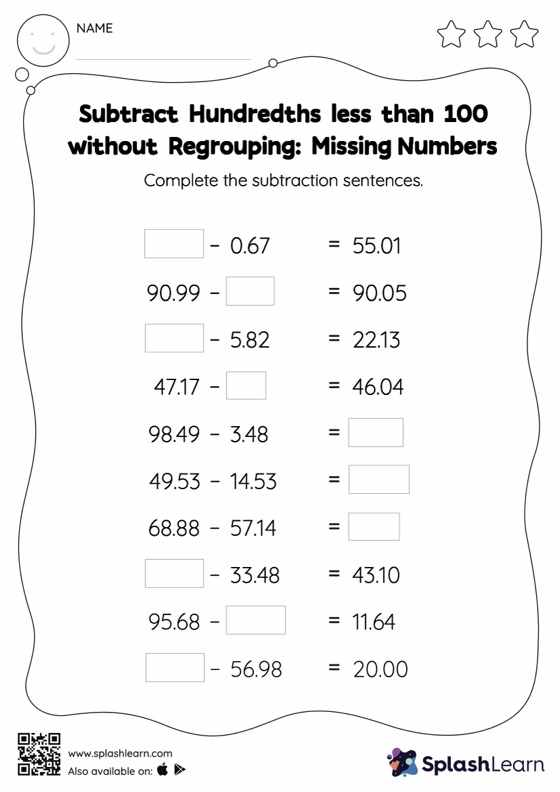# Subtract Hundredths less than 100 without Regrouping: Missing Numbers Worksheet

Home > Subtract Hundredths less than 100 without Regrouping: Missing NumbersDoes your child know how to subtract hundredths less than 100 without regrouping? Invite them to practice this concept here. Students find the missing number while subtracting decimals by using the relationship between addition and subtraction. Subtract hundredths less than 100 without regrouping worksheet does not require them to regroup numbers.